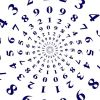#### You may also like### Digital Equation

Can you find a three digit number which is equal to the sum of the hundreds digit, the square of the tens digit and the cube of the units digit?### Euler's Totient Function

How many numbers are there less than $n$ which have no common factors with $n$?### Frosty the Snowman

Frosty the Snowman is melting. Can you use your knowledge of differential equations to find out how his volume changes as he shrinks?

# Curvy Equation

##### Age 16 to 18Challenge Level

Sketch the graph of the function $\text{h}$, where:

$$\text{h}(x) = \frac {\ln x} x, \quad (x>0)$$

Some things you could think about when sketching a graph:
• Are there any values of $x$ for which the function is undefined?
• What happens as $x$ gets really large?
• What happens as $x$ gets close to 0?
• Can you find the gradient of the function? What does this tell you?
You might want to think about these in a different order, for example knowing the gradient may help you work out how the function behaves for large (or small) $x$.

Hence, or otherwise, find all pairs of distinct positive integers $m$ and $n$ which satisfy the equation:$$n^m=m^n$$

"Hence" means that the previous part of the question should be useful in some way.
Does the function $\text{h}(x)=\frac {\ln x} x$ suggest anything you could do to the equation $n^m=m^n$?
Can you rearrange the equation so that $n$ is on one side and $m$ is on the other?
If you wanted to find two values such that $\text{f}(a) = \text{f} (b)$, how could you use a graph of $y=\text{f}(x)$ to help?

STEP Mathematics I, 1988, Q1. Question reproduced by kind permission of Cambridge Assessment Group Archives. The question remains Copyright University of Cambridge Local Examinations Syndicate ("UCLES"), All rights reserved.